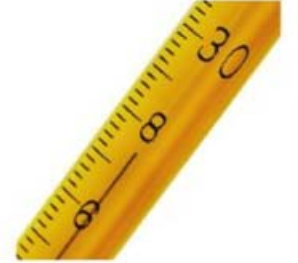# Problem: Read each measurement to the correct number of significant figures. Note: Laboratory glassware should always be read from the bottom of the meniscus. Digital balances normally display mass to the correct number of significant figures for that particular balance.

###### FREE Expert Solution
93% (472 ratings)
###### Problem Details

Read each measurement to the correct number of significant figures. Note: Laboratory glassware should always be read from the bottom of the meniscus. Digital balances normally display mass to the correct number of significant figures for that particular balance.What scientific concept do you need to know in order to solve this problem?

Our tutors have indicated that to solve this problem you will need to apply the Significant Figures: Precision in Measurements concept. You can view video lessons to learn Significant Figures: Precision in Measurements. Or if you need more Significant Figures: Precision in Measurements practice, you can also practice Significant Figures: Precision in Measurements practice problems.

What is the difficulty of this problem?

Our tutors rated the difficulty ofRead each measurement to the correct number of significant f...as low difficulty.

How long does this problem take to solve?

Our expert Chemistry tutor, Jules took 1 minute and 27 seconds to solve this problem. You can follow their steps in the video explanation above.

What professor is this problem relevant for?

Based on our data, we think this problem is relevant for Professor Mack's class at CSUS.

What textbook is this problem found in?

Our data indicates that this problem or a close variation was asked in Chemistry: A Molecular Approach - Tro 2nd Edition. You can also practice Chemistry: A Molecular Approach - Tro 2nd Edition practice problems.